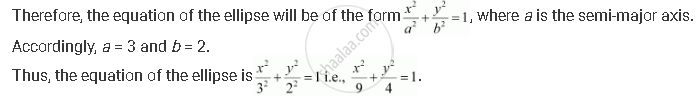CBSE (Arts) Class 11CBSE
Share

# Find the Equation for the Ellipse that Satisfies the Given Conditions: Ends of Major Axis (±3, 0), Ends of Minor Axis (0, ±2) - CBSE (Arts) Class 11 - Mathematics

ConceptEllipse Standard Equations of an Ellipse

#### Question

Find the equation for the ellipse that satisfies the given conditions: Ends of major axis (±3, 0), ends of minor axis (0, ±2)

#### Solution

Ends of major axis (±3, 0), ends of minor axis (0, ±2)

Here, the major axis is along the x-axisIs there an error in this question or solution?

#### Video TutorialsVIEW ALL 

Solution Find the Equation for the Ellipse that Satisfies the Given Conditions: Ends of Major Axis (±3, 0), Ends of Minor Axis (0, ±2) Concept: Ellipse - Standard Equations of an Ellipse.
S